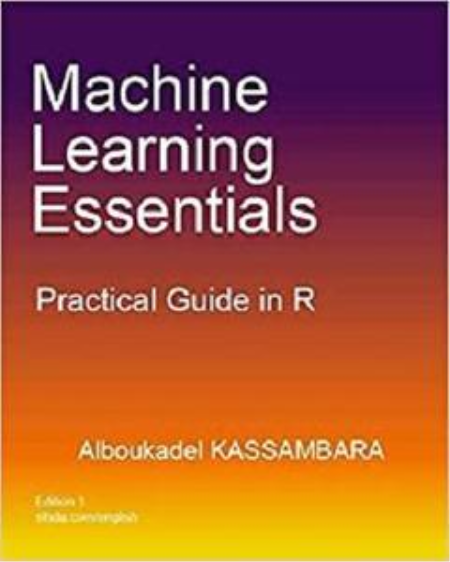English | ISBN: 1986406857 | 210 pages | EPUB | March 10, 2018 | 1.51 Mb

Discovering knowledge from big multivariate data, recorded every days, requires specialized machine learning techniques.

This book presents an easy to use practical guide in R to compute the most popular machine learning methods for exploring real word data sets, as well as, for building predictive models.

The main parts of the book include: A) Unsupervised learning methods, to explore and discover knowledge from a large multivariate data set using clustering and principal component methods. You will learn hierarchical clustering, k-means, principal component analysis and correspondence analysis methods. B) Regression analysis, to predict a quantitative outcome value using linear regression and non-linear regression strats. C) Classification techniques, to predict a qualitative outcome value using logistic regression, discriminant analysis, naive bayes classifier and support vector machines. D) Advanced machine learning methods, to build robust regression and classification models using k-nearest neighbors methods, decision tree models, ensemble methods (bagging, random forest and boosting). E) Model selection methods, to select automatically the best combination of predictor variables for building an optimal predictive model. These include, best subsets selection methods, stepwise regression and penalized regression (ridge, lasso and elastic net regression models). We also present principal component-based regression methods, which are useful when the data contain multiple correlated predictor variables. F) Model validation and evaluation techniques for measuring the performance of a predictive model. G) Model diagnostics for detecting and fixing a potential problems in a predictive model. The book presents the basic principles of these tasks and provide many examples in R. This book offers solid guidance in data mining for students and researchers.

Key features:

Covers machine learning algorithm and implementation

Key mathematical concepts are presented

Short, self-contained chapters with practical examples.